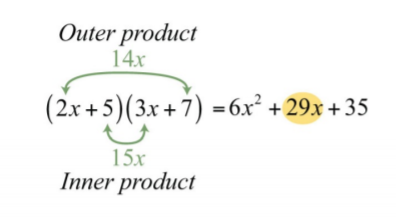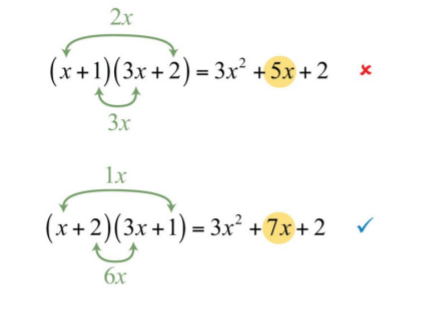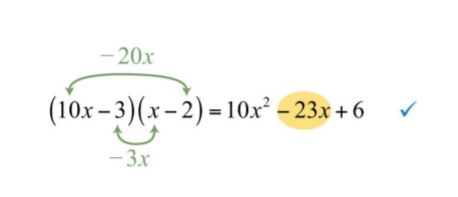# 6.3: Factoring Trinomials of the Form ax²+bx+c

•• Anonymous
• LibreTexts
$$\newcommand{\vecs}{\overset { \rightharpoonup} {\mathbf{#1}} }$$ $$\newcommand{\vecd}{\overset{-\!-\!\rightharpoonup}{\vphantom{a}\smash {#1}}}$$$$\newcommand{\id}{\mathrm{id}}$$ $$\newcommand{\Span}{\mathrm{span}}$$ $$\newcommand{\kernel}{\mathrm{null}\,}$$ $$\newcommand{\range}{\mathrm{range}\,}$$ $$\newcommand{\RealPart}{\mathrm{Re}}$$ $$\newcommand{\ImaginaryPart}{\mathrm{Im}}$$ $$\newcommand{\Argument}{\mathrm{Arg}}$$ $$\newcommand{\norm}{\| #1 \|}$$ $$\newcommand{\inner}{\langle #1, #2 \rangle}$$ $$\newcommand{\Span}{\mathrm{span}}$$ $$\newcommand{\id}{\mathrm{id}}$$ $$\newcommand{\Span}{\mathrm{span}}$$ $$\newcommand{\kernel}{\mathrm{null}\,}$$ $$\newcommand{\range}{\mathrm{range}\,}$$ $$\newcommand{\RealPart}{\mathrm{Re}}$$ $$\newcommand{\ImaginaryPart}{\mathrm{Im}}$$ $$\newcommand{\Argument}{\mathrm{Arg}}$$ $$\newcommand{\norm}{\| #1 \|}$$ $$\newcommand{\inner}{\langle #1, #2 \rangle}$$ $$\newcommand{\Span}{\mathrm{span}}$$$$\newcommand{\AA}{\unicode[.8,0]{x212B}}$$

Learning Objectives

• Factor trinomials of the form $$ax^{2}+bx+c$$.
• Factor trinomials with a common factor.

## Factoring Trinomials of the Form $$ax^{2}+bx+c$$

Factoring trinomials of the form $$ax^{2}+bx+c$$ can be challenging because the middle term is affected by the factors of both $$a$$ and $$c$$. To illustrate this, consider the following factored trinomial:

$$10x^{2}+17x+3=(2x+3)(5x+1)$$

We can multiply to verify that this is the correct factorization.

\begin{aligned} (2x+3)(5x+1)&=10x^{2}+2x+15x+3 \\ &=10x^{2}+17x+3\quad\color{Cerulean}{\checkmark} \end{aligned}

As we have seen before, the product of the first terms of each binomial is equal to the first term of the trinomial. The middle term of the trinomial is the sum of the products of the outer and inner terms of the binomials. The product of the last terms of each binomial is equal to the last term of the trinomial. Visually, we have the following:Figure $$\PageIndex{1}$$

In general,

\begin{aligned} \color{Cerulean}{a}\color{black}{x^{2}+}\color{Cerulean}{b}\color{black}{x+}\color{Cerulean}{c}&= (px+m)(qx+n) \\ &=pqx^{2}+pnx+qmx+mn \\ &=\color{Cerulean}{pq}\color{black}{x^{2}+}\color{Cerulean}{(pn+qm)}\color{black}{x+}\color{Cerulean}{mn} \end{aligned}

This gives us,

$a=pq\quad\text{and}\quad b=pn+qm,\quad\text{where}\quad c=mn$

In short, when the leading coefficient of a trinomial is something other than $$1$$, there will be more to consider when determining the factors using the trial and error method. The key lies in the understanding of how the middle term is obtained. Multiply $$(2x+5)(3x+7)$$ and carefully follow the formation of the middle term.

$$\begin{array}{ccc} {(\color{Cerulean}{2x}\color{black}{+}\color{OliveGreen}{5}\color{black}{)(3x+7)=\color{Cerulean}{2x}\color{black}{\cdot 3x}}}&{\underbrace{+\color{Cerulean}{2x}\color{black}{\cdot 7+}\color{OliveGreen}{5}\color{black}{\cdot 3x}}}&{+\color{OliveGreen}{5}\color{black}{\cdot 7}} \\ {}&{\color{Cerulean}{middle\:term}}&{} \end{array}$$

\begin{aligned} &=6x^{2}+14x+15x+35 \\ &=6x^{2}+29x+35 \end{aligned}

If we think of the FOIL method for multiplying binomials, then the middle term results from the sum of the inner product and the outer product. In this case, $$14x+15x=29x$$, as illustrated below:Figure $$\PageIndex{2}$$

For this reason, we need to look for products of the factors of the first and last terms whose sum is equal to the coefficient of the middle term. For example, to factor $$6x^{2}+29x+35$$, look at the factors of $$6$$ and $$35$$.

$$\begin{array}{ccc}{6=1\cdot 6}&{\quad}&{35=1\cdot 35}\\{=\color{OliveGreen}{2\cdot 3}}&{\quad}&{=\color{OliveGreen}{5\cdot 7}} \end{array}$$

The combination that produces the coefficient of the middle term is $$2⋅7+3⋅5=14+15=29$$. Make sure that the outer terms have coefficients $$2$$ and $$7$$, and that the inner terms have coefficients $$5$$ and $$3$$. Use this information to factor the trinomial:

\begin{aligned} 6x^{2}+29x+35&=(2x\quad\color{Cerulean}{?}\color{black}{)(3x}\quad\color{Cerulean}{?}\color{black}{)} \\ &=(2x+5)(3x+7) \end{aligned}

Example $$\PageIndex{1}$$

Factor:

$$3x^{2}+7x+2$$.

Solution:

Since the leading coefficient and the last term are both prime, there is only one way to factor each.

$$3=1\cdot 3\quad\text{and}\quad 2=1\cdot 2$$

Begin by writing the factors of the first term, $$3x^{2}$$, as follows:

$$3x^{2}+7x+2=(x\quad\color{Cerulean}{?}\color{black}{)(3x}\quad\color{Cerulean}{?}\color{black}{)}$$

The middle and last term are both positive; therefore, the factors of $$2$$ are chosen as positive numbers. In this case, the only choice is in which grouping to place these factors.

$$(x+1)(3x+2)\quad\text{or}\quad (x+2)(3x+1)$$

Determine which grouping is correct by multiplying each expression.

\begin{aligned} (x+1)(3x+2)&=3x^{2}+2x+3x+2 \\ &=3x^{2}+5x+2\quad\color{red}{x}\\(x+2)(3x+1)&=3x^{2}+x+6x+2 \\ &=3x^{2}+7x+2\quad\color{Cerulean}{\checkmark} \end{aligned}

Notice that these products differ only in their middle terms. Also, notice that the middle term is the sum of the inner and outer product, as illustrated below:Figure $$\PageIndex{3}$$

$$(x+2)(3x+1)$$

Example $$\PageIndex{2}$$

Factor:

$$12x^{2}+38x+20$$.

Solution:

First, consider the factors of the first and last terms.

$$\begin{array}{ccc}{12=1\cdot 12}&{\quad}&{20=1\cdot 20}\\{=2\cdot 6}&{\quad}&{=2\cdot 10}\\{=3\cdot 4}&{\quad}&{=4\cdot 5} \end{array}$$

We search for products of factors whose sum equals the coefficient of the middle term, $$38$$. For brevity, the thought process is illustrated starting with the factors $$2$$ and $$6$$. Factoring begins at this point with the first term.

$$12x^{2}+38x+20=(2x\quad\color{Cerulean}{?}\color{black}{)(6x}\quad\color{Cerulean}{?}\color{black}{)}$$

We search for factors of 20 that along with the factors of 12 produce a middle term of 38x

$$\begin{array}{lll} {Factors\:of\:20}&{Possible}&{factorization}\\{\color{Cerulean}{1\cdot 20}}&{(2x+1)(6x+20)}&{\color{Cerulean}{middle\:term\Rightarrow 46x}}\\{\color{Cerulean}{1\cdot 20}}&{(2x+20)(6x+1)}&{\color{Cerulean}{middle\:term\Rightarrow 122x}}\\{\color{Cerulean}{2\cdot 10}}&{(2x+2)(6x+10)}&{\color{Cerulean}{middle\:term\Rightarrow 32x}}\\{\color{Cerulean}{2\cdot 10}}&{(2x+10)(6x+2)}&{\color{Cerulean}{middle\:term\Rightarrow 64x}}\\{\color{Cerulean}{4\cdot 5}}&{(2x+4)(6x+5)}&{\color{Cerulean}{middle\:term\Rightarrow 34x}}\\{\color{Cerulean}{4\cdot 5}}&{\color{OliveGreen}{(2x+5)(6x+4)}}&{\color{OliveGreen}{middle\:term\Rightarrow 38x}\quad\color{Cerulean}{\checkmark}} \end{array}$$

Here the last combination produces a middle term of $$38x$$.Figure $$\PageIndex{4}$$

$$(2x+5)(6x+4)$$

Example $$\PageIndex{3}$$

Factor:

$$10x^{2}−23x+6$$.

Solution

First, consider the factors of the first and last terms.

$$\begin{array}{ccc}{10=1\cdot 10}&{\quad}&{6=1\cdot 6}\\{=2\cdot 5}&{\quad}&{=2\cdot 3} \end{array}$$

We are searching for products of factors whose sum equals the coefficient of the middle term, $$−23$$. Factoring begins at this point with two sets of blank parentheses:

$$10x^{2}-23x+6=(\quad )(\quad )$$

Since the last term is positive and the middle term is negative, we know that both factors of the last term must be negative. Here we list all possible combinations with the factors of $$10x^{2}=2x⋅5x$$.

$$10x^{2}-23x+6=(2x\quad\color{Cerulean}{?}\color{black}{)(5x}\quad\color{Cerulean}{?}\color{black}{)}$$

$$\begin{array}{ll}{(2x-1)(5x-6)}&{\color{Cerulean}{middle\:term\Rightarrow -17x}}\\{(2x-6)(5x-1)}&{\color{Cerulean}{middle\:term\Rightarrow -32x}}\\{(2x-2)(5x-3)}&{\color{Cerulean}{middle\:term\Rightarrow -16x}}\\{(2x-3)(5x-2)}&{\color{Cerulean}{middle\:term\Rightarrow -19x}} \end{array}$$

There is no combination that produces a middle term of $$−23x$$. We then move on to the factors of $$10x^{2}=10x⋅x$$ and list all possible combinations:

$$10x^{2}-23x+6=(10x\quad\color{Cerulean}{?}\color{black}{)(x}\quad\color{Cerulean}{?}\color{black}{)}$$

$$\begin{array}{ll}{(10x-1)(x-6)}&{\color{Cerulean}{middle\:term\Rightarrow -61x}}\\{(10x-6)(x-1)}&{\color{Cerulean}{middle\:term\Rightarrow -162x}}\\{(10x-2)(x-3)}&{\color{Cerulean}{middle\:term\Rightarrow -32x}}\\{\color{OliveGreen}{(10x-3)(x-2)}}&{\color{OliveGreen}{middle\:term\Rightarrow -23x}\quad\color{Cerulean}{\checkmark}} \end{array}$$

And we can writeFigure $$\PageIndex{5}$$

$$(10x-3)(x-2)$$. The complete check is left to the reader.

We can reduce much of the guesswork involved in factoring trinomials if we consider all of the factors of the first and last terms and their products.

Example $$\PageIndex{4}$$

Factor:

$$5x^{2}+38x-16$$.

Solution:

We begin with the factors of $$5$$ and $$16$$.

$$\begin{array}{cc}{}&{16=1\cdot 16}\\{5=1\cdot 5}&{=2\cdot 8}\\{}&{=4\cdot 4} \end{array}$$

Since the leading coefficient is prime, we can begin with the following:

$$5x^{2}+38x-16=(x\quad\color{Cerulean}{?}\color{black}{)(5x}\quad\color{Cerulean}{?}\color{black}{)}$$

We look for products of the factors of 5 and 16 that could possibly add to 38.

$$\begin{array}{lll}{Factors\:of\:16}&{Possible}&{products}\\{\color{Cerulean}{1\cdot 16}}&{1\cdot\color{Cerulean}{1}\:\color{black}{and\: 5}\cdot\color{Cerulean}{16}}&{\color{Cerulean}{products\Rightarrow\:1\:and\:80}}\\{\color{Cerulean}{1\cdot 16}}&{1\cdot \color{Cerulean}{16}\:\color{black}{and\:5}\cdot\color{Cerulean}{1}}&{\color{Cerulean}{products\Rightarrow\:16\:and\:5}}\\{\color{Cerulean}{2\cdot 8}}&{1\cdot\color{Cerulean}{2}\:\color{black}{and\:5}\cdot\color{Cerulean}{8}}&{\color{OliveGreen}{products\Rightarrow\:2\:and\:40}\quad\color{Cerulean}{\checkmark}}\\{\color{Cerulean}{2\cdot 8}}&{1\cdot\color{Cerulean}{8}\:\color{black}{and\:5}\cdot\color{Cerulean}{2}}&{\color{Cerulean}{products\Rightarrow\:8\:and\:10}}\\{\color{Cerulean}{4\cdot 4}}&{1\cdot\color{Cerulean}{4}\:\color{black}{and\:5}\cdot\color{Cerulean}{4}}&{\color{Cerulean}{products\Rightarrow\:4\:and\:20}} \end{array}$$

Since the last term is negative, we must look for factors with opposite signs. Here we can see that the products 2 and 40 add up to 38 if they have opposite signs:

$$1\cdot (\color{Cerulean}{-2}\color{black}{)+5\cdot}\color{Cerulean}{8}\color{black}{=-2+40=38}$$

Therefore, use $$−2$$ and $$8$$ as the factors of $$16$$, making sure that the inner and outer products are $$−2x$$ and $$40x$$:Figure $$\PageIndex{6}$$

$$(x+8)(5x-2)$$. The complete check is left to the reader.

After lots of practice, the process described in the previous example can be performed mentally.

Exercise $$\PageIndex{1}$$

Factor:

$$12x^{2}-31x-30$$

$$(3x-10)(4x+3)$$

When given trinomials with multiple variables, the process is similar.

Example $$\PageIndex{5}$$

Factor:

$$9x^{2}+30xy+25y^{2}$$.

Solution:

Search for factors of the first and last terms such that the sum of the inner and outer products equals the middle term.

$$\begin{array}{cc}{9x^{2}=1x\cdot 9x}&{25y^{2}=1y\cdot 25y}\\{=3x\cdot 3x}&{=5y\cdot 5y} \end{array}$$

Add the following products to obtain the middle term: $$3x⋅5y+3x⋅5y=30xy$$.

\begin{aligned} 9x^{2}+30xy+25y^{2}&=(3x\quad )(3x\quad ) \\ &=(3x+5y)(3x+5y) \\ &=(3x+5y)^{2} \end{aligned}

In this example, we have a perfect square trinomial. Check.

\begin{aligned} (3x+5y)^{2}&= 9x^{2}+2\cdot 3x\cdot 5y+25y^{2} \\ &=9x^{2}+30xy+25y^{2}\quad\color{Cerulean}{\checkmark} \end{aligned}

$$(3x+5y)^{2}$$

Exercise $$\PageIndex{2}$$

Factor:

$$16x^{2}−24xy+9y^{2}$$.

$$(4x-3y)^{2}$$

## Factoring Trinomials with Common Factors

It is a good practice to first factor out the GCF, if there is one. Doing this produces a trinomial factor with smaller coefficients. As we have seen, trinomials with smaller coefficients require much less effort to factor. This commonly overlooked step is worth identifying early.

Example $$\PageIndex{6}$$

Factor:

$$12x^{2}-27x+6$$.

Solution:

Begin by factoring out the GCF.

$$12x^{2}-27x+6=3(4x^{2}-9x+2)$$

After factoring out 3, the coefficients of the resulting trinomial are smaller and have fewer factors.

$$\begin{array}{cc}{4=\color{OliveGreen}{1\cdot 4}}&{2=\color{OliveGreen}{1\cdot 2}}\\{=2\cdot 2}&{}\end{array}$$

After some thought, we can see that the combination that gives the coefficient of the middle term is $$4(−2)+1(−1)=−8−1=−9$$.

\begin{aligned}3(4x^{2}-9x+2)&=3(4x\quad\color{Cerulean}{?}\color{black}{)(x}\quad\color{Cerulean}{?}\color{black}{)} \\ &=3(4x-1)(x-2) \end{aligned}

Check.

\begin{aligned} 3(4x-1)(x-2)&=3(4x^{2}-8x-x+2) \\ &=3(4x^{2}-9x+2) \\ &=12x^{2}-27x+6\quad\color{Cerulean}{\checkmark} \end{aligned}

The factor $$3$$ is part of the factored form of the original expression; be sure to include it in the answer.

$$3(4x-1)(x-2)$$

It is a good practice to consistently work with trinomials where the leading coefficient is positive.

Example $$\PageIndex{7}$$

Factor:

$$−x^{2}+2x+15$$.

Solution

In this example, the leading coefficient is $$−1$$. Before beginning the factoring process, factor out the $$−1$$:

$$-x^{2}+2x+15=-1(x^{2}-2x-15)$$

At this point, factor the remaining trinomial as usual, remembering to write the $$−1$$ as a factor in your final answer. Because $$3 + (−5) = −2$$, use $$3$$ and $$5$$ as the factors of $$15$$.

\begin{aligned} -x^{2}+2x=15&=-1(x^{2}-2x-15) \\ &=-1(x\quad )(x\quad )\\ &=-(x+3)(x-5) \end{aligned}

$$-1(x+3)(x-5)$$. The check is left to the reader.

Example $$\PageIndex{8}$$

Factor:

$$-60a^{2}-5a+30$$

Solution

The GCF of all the terms is $$5$$. However, in this case factor out $$−5$$ because this produces a trinomial factor where the leading coefficient is positive.

$$-60a^{2}-5a+30=-5(12a^{2}+a-6)$$

Focus on the factors of $$12$$ and $$6$$ that combine to give the middle coefficient, $$1$$.

$$\begin{array}{cc}{12=1\cdot 12}&{6=1\cdot 6}\\{=2\cdot 6}&{=\color{OliveGreen}{2\cdot 3}}\\{=\color{OliveGreen}{3\cdot 4}}&{} \end{array}$$

After much thought, we find that $$3⋅3−4⋅2=9−8=1$$. Factor the remaining trinomial.

\begin{aligned} -60a^{2}-5a+30&=-5(12a^{2}+a-6) \\ &=-5(4a\quad )(3a\quad )\\&=-5(4a+3)(3a-2) \end{aligned}

$$-5(4a+3)(3a-2)$$. The check is left to the reader.

Exercise $$\PageIndex{3}$$

Factor:

$$24+2x−x^{2}$$.

$$−1(x−6)(x+4)$$

## Factoring Using the AC Method

In this section, we factor trinomials of the form $$ax^{2}+bx+c$$ using the AC method described previously.

Example $$\PageIndex{9}$$

Factor using the AC method:

$$18x^{2}−21x+5$$.

Solution:

Here $$a = 18, b = −21$$, and $$c = 5$$.

\begin{aligned}ac&=18(5) \\ &=90 \end{aligned}

Factor $$90$$ and search for factors whose sum is $$−21$$.

\begin{aligned} 90&=1(90) \\ &=2(45) \\ &=3(30) \\ &=5(18) \\ &=\color{OliveGreen}{6(15)}\quad\color{Cerulean}{\checkmark} \\ &=9(10) \end{aligned}

In this case, the sum of the factors $$−6$$ and $$−15$$ equals the middle coefficient, $$−21$$. Therefore, $$−21x=−6x−15x$$, and we can write

$$18x^{2}\color{OliveGreen}{-21x}\color{black}{+5=18x^{2}}\color{OliveGreen}{-6x-15x}\color{black}{+5}$$

Factor the equivalent expression by grouping.

\begin{aligned} 18x^{2}-21x+5&=18x^{2}-6x-15x+5 \\ &=6x(3x-1)-5(3x-1) \\ &=(3x-1)(6x-5) \end{aligned}

$$(3x-1)(6x-5)$$

Example $$\PageIndex{10}$$

Factor using the AC method: $$9x^{2}−61x−14$$.

Solution:

Here $$a = 9, b = −61$$, and $$c = −14$$.

We factor $$-126$$ as follows:

\begin{aligned} -126&=1(-126) \\ &=\color{OliveGreen}{2(-63)}\quad\color{Cerulean}{\checkmark} \\ &=3(-42)\\&=6(-21)\\&=7(-18)\\&=9(-14) \end{aligned}

The sum of factors $$2$$ and $$−63$$ equals the middle coefficient, $$−61$$. Replace $$−61x$$ with $$2x−63x$$:

\begin{aligned} 9x^{2}-61x-14&=9x^{2}+2x-63x-14\quad\color{Cerulean}{Rearrange\:the\:terms.} \\ &=9x^{2}-63x+2x-14\quad\color{Cerulean}{Factor\:by\:grouping.}\\&=9x(x-7)+2(x-7) \\ &=(x-7)(9x+2) \end{aligned}

$$(x-7)(9x+2)$$. The check is left to the reader.

## Key Takeaways

• If a trinomial of the form $$ax^{2}+bx+c$$ factors into the product of two binomials, then the coefficient of the middle term will be the sum of certain products of factors of the first and last terms.
• If the trinomial has a greatest common factor, then it is a best practice to first factor out the GCF before attempting to factor it into a product of binomials.
• If the leading coefficient of a trinomial is negative, then it is a best practice to factor that negative factor out before attempting to factor the trinomial.
• Factoring trinomials of the form $$ax^{2}+bx+c$$ takes lots of practice and patience. It is extremely important to take the time to become proficient by working lots of exercises.

Exercise $$\PageIndex{4}$$ Factoring Trinomials

Factor.

1. $$3x^{2}−14x−5$$
2. $$5x^{2}+7x+2$$
3. $$2x^{2}+5x−3$$
4. $$2x^{2}+13x−7$$
5. $$2x^{2}+9x−5$$
6. $$7x^{2}+20x−3$$
7. $$7x^{2}−46x−21$$
8. $$3x^{2}+x−2$$
9. $$5x^{2}+34x−7$$
10. $$5x^{2}−28x−12$$
11. $$9x^{2}−12x+4$$
12. $$4x^{2}−20x+25$$
13. $$49x^{2}+14x+1$$
14. $$25x^{2}−10x+1$$
15. $$2x^{2}+7x+16$$
16. $$6x^{2}−19x−10$$
17. $$27x^{2}+66x−16$$
18. $$12x^{2}−88x−15$$
19. $$12y^{2}−8y+1$$
20. $$16y^{2}−66y−27$$
21. $$9x^{2}−12xy+4y^{2}$$
22. $$25x^{2}+40x+16$$
23. $$15x^{2}−26xy+8y^{2}$$
24. $$12a^{2}−4ab−5b^{2}$$
25. $$4x^{2}y^{2}+16xy−9$$
26. $$20x^{2}y^{2}+4xy−7$$
27. The area of a rectangle is given by the function $$A(x)=3x^{2}−10x+3$$, where $$x$$ is measured in meters. Rewrite this function in factored form.
28. The area of a rectangle is given by the function $$A(x)=10x^{2}−59x−6$$, where $$x$$ is measured in meters. Rewrite this function in factored form.

1. $$(x−5)(3x+1)$$

3. $$(x+3)(2x−1)$$

5. $$(x+5)(2x−1)$$

7. $$(x−7)(7x+3)$$

9. $$(x+7)(5x−1)$$

11. $$(3x−2)^{2}$$

13. $$(7x+1)^{2}$$

15. Prime

17. $$(3x+8)(9x−2)$$

19. $$(6y−1)(2y−1)$$

21. $$(3x−2y)^{2}$$

23. $$(3x−4y)(5x−2y)$$

25. $$(2xy−1)(2xy+9)$$

27. $$A(x)=(3x−1)(x−3)$$

Exercise $$\PageIndex{5}$$ Factoring Trinomials with Common Factors

Factor.

1. $$6x^{2}−20x−16$$
2. $$45x^{2}+27x−18$$
3. $$20x^{2}−20x+5$$
4. $$3x^{2}+39x−90$$
5. $$16x^{2}+26x−10$$
6. $$54x^{2}−15x+6$$
7. $$45x^{2}−45x−20$$
8. $$90x^{2}+300x+250$$
9. $$40x^{2}−36xy+8y^{2}$$
10. $$24a^{2}b^{2}+18ab−81$$
11. $$6x^{2}y^{2}+46xy+28$$
12. $$2x^{5}+44x^{4}+144x^{3}$$
13. $$5x^{3}−65x^{2}+60x$$
14. $$15a^{4}b^{2}−25a^{3}b−10a^{2}$$
15. $$6a^{4}b+2a^{3}b^{2}−4a^{2}b^{3}$$
16. $$20a^{3}b^{2}−60a^{2}b^{3}+45ab^{4}$$

1. $$2(x−4)(3x+2)$$

3. $$5(2x−1)^{2}$$

5. $$2(8x^{2}+13x−5)$$

7. $$5(3x−4)(3x+1)$$

9. $$4(5x−2y)(2x−y)$$

11. $$2(xy+7)(3xy+2)$$

13. $$5x(x−12)(x−1)$$

15. $$2a^{2}b(3a−2b)(a+b)$$

Exercise $$\PageIndex{6}$$ Factoring Trinomials with Common Factors

Factor out $$−1$$ and then factor further.

1. $$−x^{2}−4x+21$$
2. $$−x^{2}+x+12$$
3. $$−x^{2}+15x−56$$
4. $$−x^{2}+x+72$$
5. $$−y^{2}+10y−25$$
6. $$−y^{2}−16y−64$$
7. $$36−9a−a^{2}$$
8. $$72−6a−a^{2}$$
9. $$32+4x−x^{2}$$
10. $$200+10x−x^{2}$$

1. $$−1(x−3)(x+7)$$

3. $$−1(x−7)(x−8)$$

5. $$−1(y−5)^{2}$$

7. $$−1(a−3)(a+12)$$

9. $$−1(x−8)(x+4)$$

Exercise $$\PageIndex{7}$$ Factoring Trinomials with Common Factors

Factor out a negative common factor first and then factor further if possible.

1. $$−8x^{2}+6x+9$$
2. $$−4x^{2}+28x−49$$
3. $$−18x^{2}−6x+4$$
4. $$2+4x−30x^{2}$$
5. $$15+39x−18x^{2}$$
6. $$90+45x−10x^{2}$$
7. $$−2x^{2}+26x+28$$
8. $$−18x^{3}−51x^{2}+9x$$
9. $$−3x^{2}y^{2}+18xy^{2}−24y^{2}$$
10. $$−16a^{4}+16a^{3}b−4a^{2}b^{2}$$
11. The height in feet of a projectile launched from a tower is given by the function $$h(t)=−16t^{2}+64t+80$$, where $$t$$ represents the number of seconds after launch. Rewrite the given function in factored form.
12. The height in feet of a projectile launched from a tower is given by the function $$h(t)=−16t^{2}+64t+192$$, where $$t$$ represents the number of seconds after launch. Rewrite the given function in factored form.

1. $$−(2x−3)(4x+3)$$

3. $$−2(3x−1)(3x+2)$$

5. $$−3(2x−5)(3x+1)$$

7. $$−2(x−14)(x+1)$$

9. $$−3y^{2}(x−4)(x−2)$$

11. $$h(t)=−16(t+1)(t−5)$$

Exercise $$\PageIndex{8}$$ Factoring Using the AC Method

Factor using the AC method.

1. $$2x^{2}+5x−7$$
2. $$3x^{2}+7x−10$$
3. $$4x^{2}−25x+6$$
4. $$16x^{2}−38x−5$$
5. $$6x^{2}+23x−18$$
6. $$8x^{2}+10x−25$$
7. $$4x^{2}+28x+40$$
8. $$−6x^{2}−3x+30$$
9. $$12x^{2}−56xy+60y^{2}$$
10. $$20x^{2}+80xy+35y^{2}$$

1. $$(x−1)(2x+7)$$

3. $$(x−6)(4x−1)$$

5. $$(2x+9)(3x−2)$$

7. $$4(x+2)(x+5)$$

9. $$4(x−3y)(3x−5y)$$

Exercise $$\PageIndex{9}$$ Discussion Board Topics

1. Create your own trinomial of the form $$ax^{2}+bx+c$$ that factors. Share it, along with the solution, on the discussion board.
2. Write out your own list of steps for factoring a trinomial of the form $$ax^{2}+bx+c$$ and share it on the discussion board.
3. Create a trinomial of the form $$ax^{2}+bx+c$$ that does not factor and share it along with the reason why it does not factor.